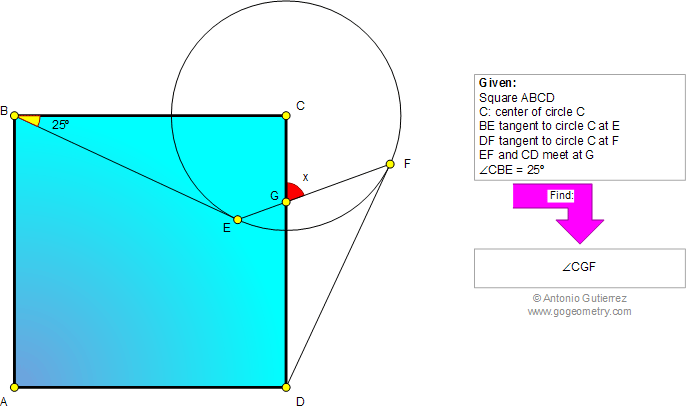# Online Geometry Problem 752: Square, Circle, Tangent, Chord, Angle. Level: High School, Honors Geometry, College, Mathematics Education

 The figure shows a square ABCD and a circle of center C. BE and DF are tangent to circle C at E and F, respectively. EF and CD meet at G. If the measure of angle CBE is 25 degrees, find the measure of angle CGF.See also: Typography of problem 752
 Home | Search | Geometry | Problems | All Problems | Open Problems | Visual Index | 751-760 | Square | Triangles | Circles | Chord | Tangent line | Congruence | Email | Solution / comment | by Antonio Gutierrez